# Characterize Battery Cell for Electric Vehicles

This example shows how to characterize a battery cell for electric vehicle applications using the test method from . This example estimates the parameters of BAK N18650CL-29 18650 type lithium-ion cells  at five different ambient temperatures. The battery hybrid pulse power characterization (HPPC) test is performed in controlled environmental chambers.

### Battery Testing

A typical HPPC data is a set of discharge-charge pulses, applied to a battery at different state of charge (SOC) and at a given temperature . The magnitude of the pulse depends upon the cell capacity and the test temperature. At the end of every sequence of discharge-charge pulse operations, the SOC decreases by about 10% by applying a constant discharge current of C/3. A long rest time of one hour is recommended for the cells to relax after every sequence of discharge-charge pulses. This process continues until it covers all points of interest in the SOC range. For more information, see .

#### Test Conditions and Setup

A Biologic BCS-815 8-channel battery tester, equipped with standard cables, accessories, and thermocouples, performed the battery HPPC tests . The test chamber maintained temperature and humidity control. To measure the temperature, the tester used the Agilent 34972A data acquisition system equipped with multiplexer capabilities (34901A 20-Channel Multiplexer). The system tested three different samples. The test was reproducible each time. The cells were tested at five different temperatures: ${0}^{\mathit{o}}\mathit{C},{10}^{\mathit{o}}\mathit{C},{25}^{\mathit{o}}\mathit{C},{35}^{\mathit{o}}\mathit{C},\mathrm{and}\text{\hspace{0.17em}}{45}^{\mathit{o}}\mathit{C}$ chamber temperatures. During the test duration, the temperature was uniform. For more information about the test procedure, see .

These plots show the battery test data. Each test data has nine pair of discharge-charge pulses. After each discharge-charge operation, a C/3 constant current (SOC sweep step) decreases the cell SOC by 10%. To visualize the battery test data, at the MATLAB Command Window, enter:

```T_vec = [0 10 25 35 45]; % temperature in degree Centigrade for i = 1:5 plotBatteryTestData(T_vec(i)); end```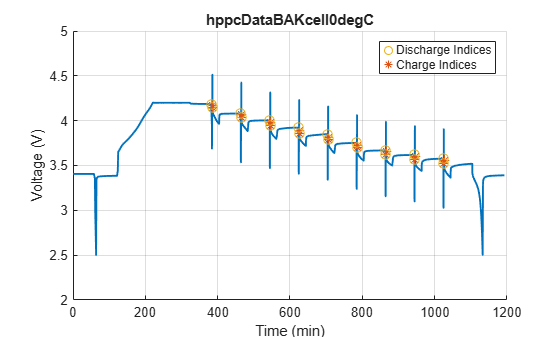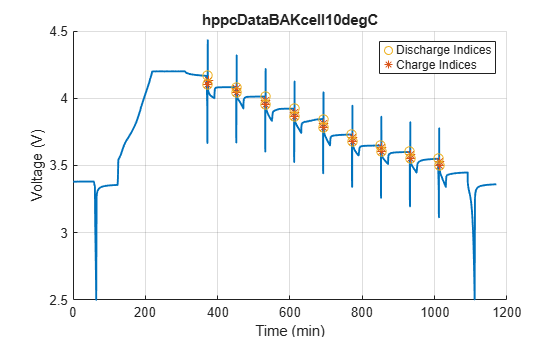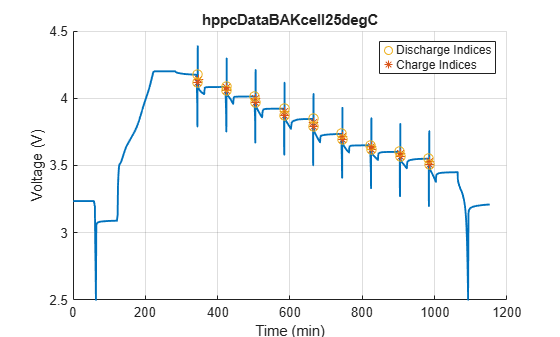### Battery Parameter Estimation

The Battery (Table-Based) block in Simscape Battery uses the equivalent circuit modeling approach. You can capture different physical phenomena of a cell by connecting multiple resistor-capacitor (RC) pairs in series. In the Battery (Table-Based) block, you can select up to five RC pairs. You can derive the value of the resistance and time constant parameters from the HPPC test data.

This equation defines the voltage response of a battery cell:

`$\mathit{V}={\mathit{V}}_{0}-\mathit{I}×{\mathit{R}}_{\mathit{o}}-\mathit{I}×\left(\sum {\mathit{R}}_{\mathit{i}}\left(1-\mathrm{exp}\left(-\frac{\mathit{t}}{{\tau }_{\mathit{i}}}\right)\right)\right),$`

where:

• ${\mathit{V}}_{0}$ is the cell open-circuit potential.

• ${\mathit{R}}_{\mathit{o}}$ is the cell ohmic resistance.

• ${\mathit{R}}_{\mathit{i}}\text{\hspace{0.17em}}\mathrm{and}\text{\hspace{0.17em}}{\tau }_{\mathit{i}}$ are the cell i-th RC pair resistance and time constant values.

• $\mathit{I}$ is the current passing through the cell.

• t is the elasped time.

All parameters are a function of the SOC and cell temperature. Since HPPC tests are typically performed at constant temperatures, you can ignore the temperature dependence in the parameter estimation. This figure shows a typical discharge-charge profile.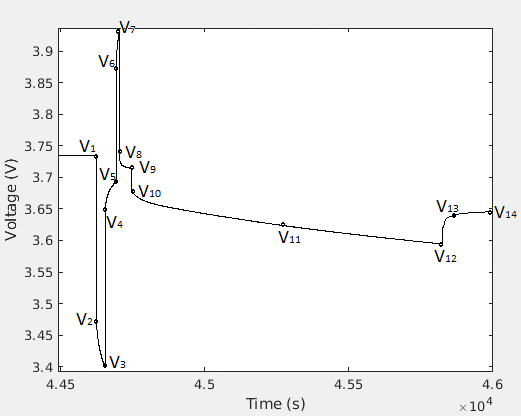You can estimate the ohmic resistance ${\mathit{R}}_{\mathit{o}}$ from the sudden voltage change at the start of a discharge or a charge pulse (for example V1 to V2 or V5 to V6). To estimate the ${\mathit{R}}_{\mathit{i}}\text{\hspace{0.17em}}\mathrm{and}\text{\hspace{0.17em}}{\tau }_{\mathit{i}}$ parameters, you can use the short voltage relaxation immediately after the discharge (V4 - V5) or the charge (V8 - V9) pulse. You can extract more time constants from the longer voltage relaxation (V12 - V14) after the SOC sweep step (V9 - V12). The `fminsearch` MATLAB function or the curvefit functions from the Curve Fitting Toolbox (TM) use the test data points to fit ${\mathit{R}}_{\mathit{i}}\text{\hspace{0.17em}}\mathrm{and}\text{\hspace{0.17em}}{\tau }_{\mathit{i}}$ parameters at different SOC points. To find the open-circuit potential at a given SOC, use the period at the end of the long relaxation (V1) and just before the discharge-charge pulse operation.

In this example, you will use the `batteryParametersLUT` function to estimate ${\mathit{V}}_{0}$, ${\mathit{R}}_{\mathit{o}}$ and ${\mathit{R}}_{\mathit{i}}-\text{\hspace{0.17em}}{\tau }_{\mathit{i}}$ parameters for the battery.

#### Parameter Estimation Method

The `batteryParametersLUT` function takes these arguments as input:

1. HPPC data, specified as a column vector of time (in seconds), current (in Ampere), voltage (in Volt), and SOC (0-1). The 4th column in the HPPC data, SOC, is optional. You must ensure that the data covers the entire SOC range for the battery, starting from a fully charged cell (SOC=1) to a nearly depleted battery cell, to avoid any extrapolation of the parameters.

2. Cell capacity and the initial SOC in the HPPC data, specified as an array of the two scalar values. This example uses the cellProp parameter to stores these values.

3. The discharge pulse current (in A), the charge pulse current (in A), the SOC sweep current (in A), and the tolerance value to detect these current specified as an array of four scalar values. You must define tolerance to a suitable value (typically 0.01-0.05) for the function to detect current inflexion points in the HPPC data. This example uses the hppcProtocol parameter to stores these values

4. Number of RC pairs specified as integer, along with their initial estimates specified as an array of scalar values, for the short (V4-V5 and V8-V9) and the long relaxation (V12-V14) curves.

5. Data fit method, specified as `fminsearch` or `curvefit`.

6. Option to enable debugging, specified as `true` or `false`. If `true`, the function plots the HPPC curve used for curve fit and the points selected for evaluating the ohmic resistance, dynamic parameters, and the open-circuit potential.

This code shows how to use the `batteryparametersLUT` function. Extracting the parameters is a two steps process:

```result = batt_BatteryCellCharacterization.batteryParametersLUT(... [time, current, voltage],... cellProp,hppcProtocol,... numPairShort, iniEstimateShort,... numPairLong, iniEstimateLong,... "fminsearch", false); % Method to define number of RC pairs and the initial estimates % numRCpairs = 1; % iniEstimateRC = [1e-3 10]; % [R1, Tau1] % numRCpairsRest = 3; % iniEstimateRCrest = [1e-3 1 5e-3 10 1e-2 100]; % [R1, Tau1, R2, Tau2, R3, Tau3] % Specify all resistance values in Ohms % Specify all time constant values in seconds battRC = exportResultsForLib(... result, ... 0:1e-3:1, ... true); ```

You must provide the `exportResultsForLib` function these input arguments:

1. The output of the `batteryParametersLUT` function.

2. A SOC range, specified as an array of SOC breakpoints. This example uses SOC breakpoints of 0:1e-3:1.

3. The plot option, specified as `true` or `false`. If `true`, the function plots all the estimated battery parameters as a function of the SOC range.

The `battRC` workspace variable stores the final parameterization data.

#### Parameterize Battery (Table-Based) Block

To parameterize the Battery (Table-Based) block and visualize the verification results for all the temperatures that you specified in the `T_vec` workspace variable, at a MATLAB Command Window, enter:

```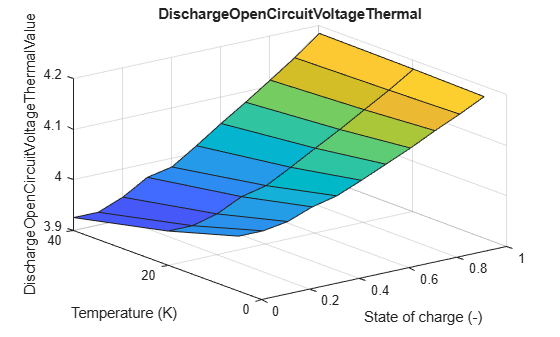optionRun = 0; % Define cell array to store parameters for different temperature cases cellParameters = cell(1,length(T_vec)); caseName = cell(1,length(T_vec));```

Estimate the battery parameters at all temperatures, as defined in the vector `T_vec`:

```for T = 1:length(T_vec) Tval = T_vec(1,T); caseName{1,T} = strcat(num2str(Tval),'degC'); disp(strcat('Estimating parameters for T = ',num2str(Tval),' degC')); % Get HPPC data for a particular temperature [time, current, voltage, cellProp, protocolHPPC] = ... getBatteryTestData(Tval); % Define the number of RC pairs to consider and the initial estimates % for resistance and the time constant values. [numRCshort, iniEstimateShort, numRClong, iniEstimateLong] = ... getBatteryIniEstimatesResTau(Tval); % Estimate battery parameters result = batt_BatteryCellCharacterization.batteryParametersLUT(... [time, current, voltage],... cellProp,protocolHPPC,... numRCshort,iniEstimateShort,... numRClong,iniEstimateLong,... "fminsearch", false); % Save generated parameters for library cellParameters{1,T} = ... exportResultsForLib(result, 0:1e-3:1, false); end```
```Estimating parameters for T =0 degC ```
```*** Number of discharge pulses =9 *** Number of charge pulses =9 *** Number of SOC sweep pulses =8 ```
```Estimating parameters for T =10 degC ```
```*** Number of discharge pulses =9 *** Number of charge pulses =9 *** Number of SOC sweep pulses =8 ```
```Estimating parameters for T =25 degC ```
```*** Number of discharge pulses =9 *** Number of charge pulses =9 *** Number of SOC sweep pulses =8 ```
```Estimating parameters for T =35 degC ```
```*** Number of discharge pulses =9 *** Number of charge pulses =9 *** Number of SOC sweep pulses =8 ```
```Estimating parameters for T =45 degC ```
```*** Number of discharge pulses =9 *** Number of charge pulses =9 *** Number of SOC sweep pulses =8 ```

Compare the long rest (relaxation) time fit against the short rest time fit to the original HPPC test data. The example runs these commands only if you select `Parameterize and Verify` in the drop-down menu above.

```% Verification Plots if optionRun for T = 1:length(T_vec) Tval = T_vec(1,T); % Get HPPC data for a particular temperature [time, current, voltage, ~, ~] = getBatteryTestData(Tval); verifyCellParam = cellParameters{1,T}; hppcCurrentData = timeseries(current,time); warning('off','all'); % Run the verification model sim('CellCharacterizationHPPC.slx'); % Post-process data and plot sim_V1 = simlog_CellCharacterizationHPPC.longRest_3RC.v.series.values; sim_V2 = simlog_CellCharacterizationHPPC.shortRest_1RC.v.series.values; sim_t = simlog_CellCharacterizationHPPC.shortRest_1RC.v.series.time; % Plot figure('Name', strcat('HPPC verification ',caseName{1,T})); plot(sim_t,sim_V1,'r--');hold on; plot(sim_t,sim_V2,'b--');hold on; plot(time,voltage,'k-');hold off; legend('Fit from long relaxation time curve', ... 'Fit from short relaxation time curve',... 'Test Data','Location','southwest'); xlabel('Time (s)'); ylabel('Voltage (V)'); title(strcat('Verification plots for T = ',num2str(Tval))); end end```

Combine the battery parameters at different temperatures into a form required by the Battery (Table Based) block.

```% Raw data generated from the parameterization paramData = cell2table(cellParameters, ... "VariableNames", caseName); % The Battery (Table-Based) block parameters % % Third argument "long" implies Battery Dynamic parameters selected based % upon the long relaxation or rest time. You can get short relaxation time % based parameters by using "short" instead of "long" in the 3rd argument % below. battModel = getBatteryTableBasedParams( ... T_vec, cellParameters, "long", ... [numRCshort numRClong]);```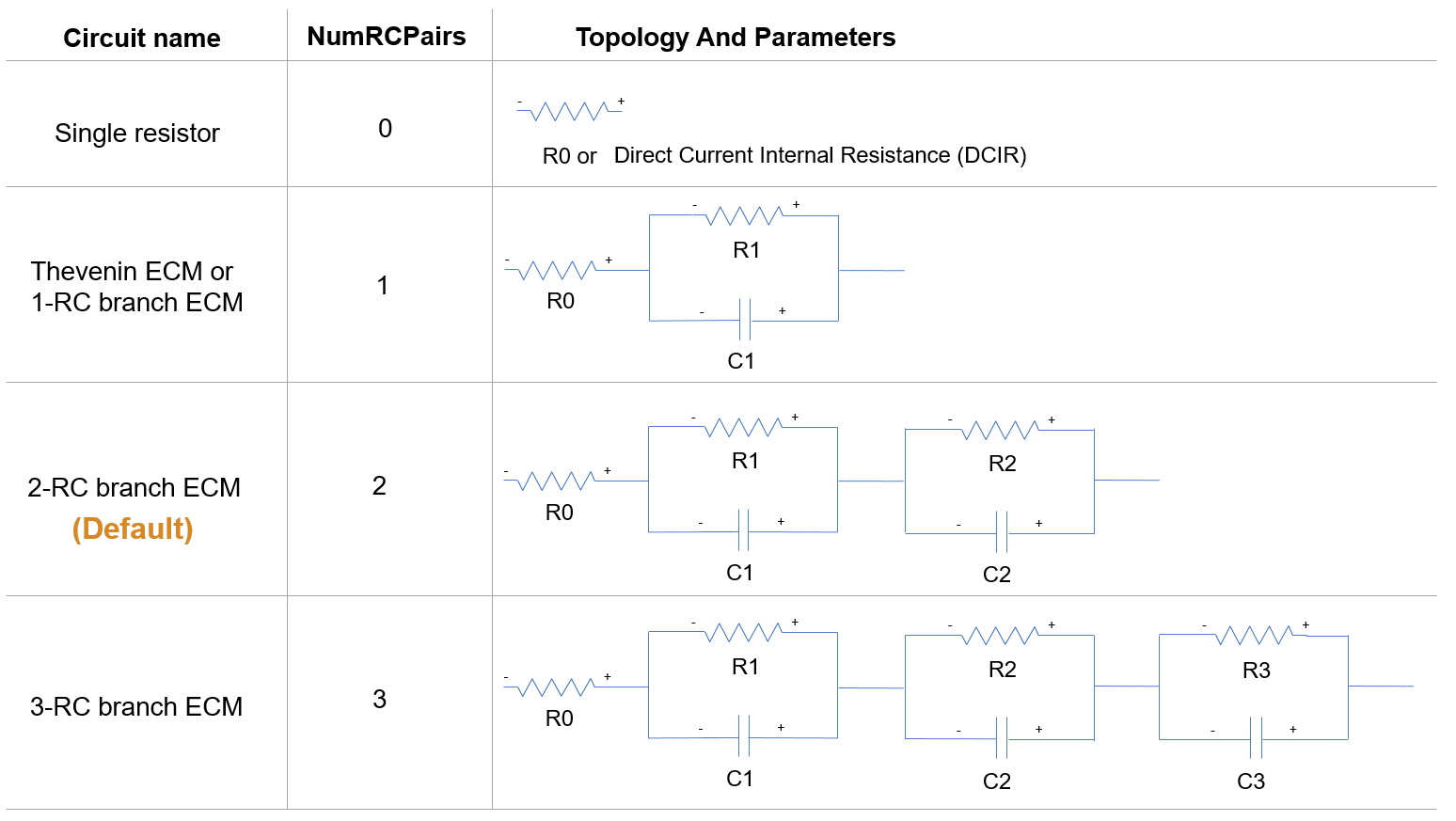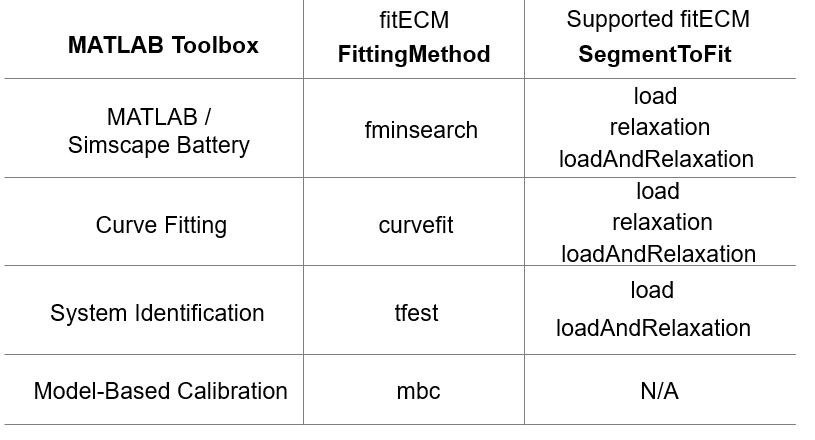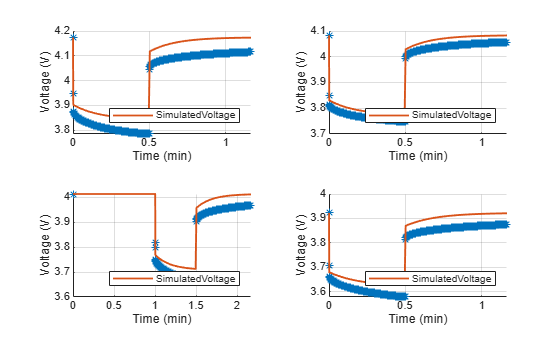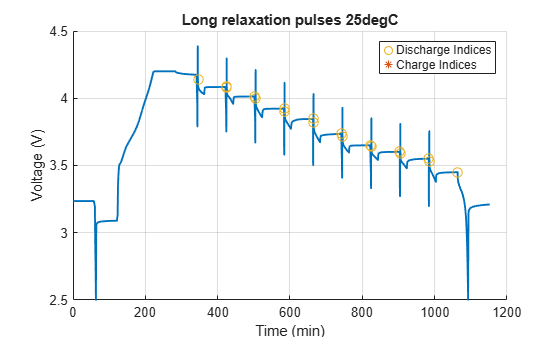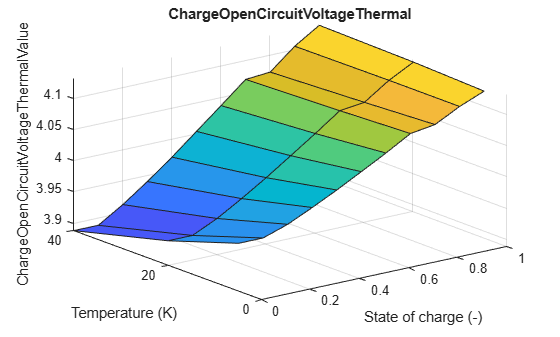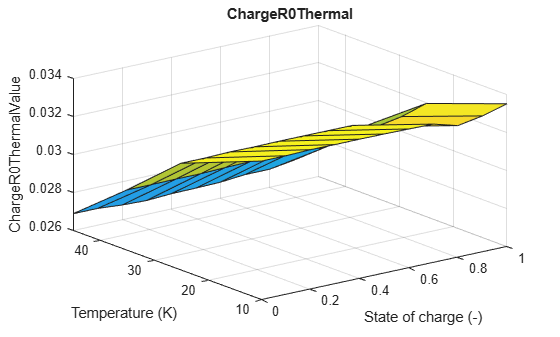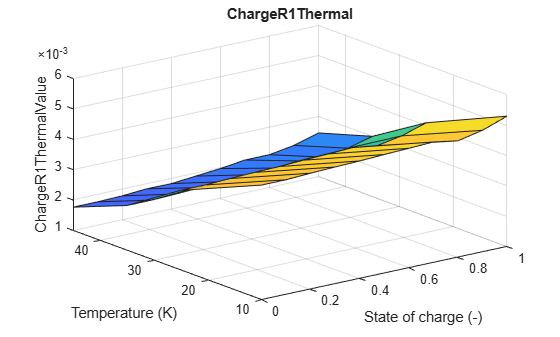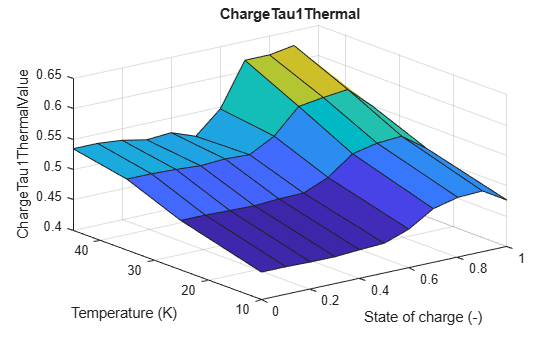The `paramData` and `battModel` workspace variables contain the raw data generated from the parameterization and the Battery (Table-Based) block parameters, respectively.

### Simulate Battery with Estimated Parameters

####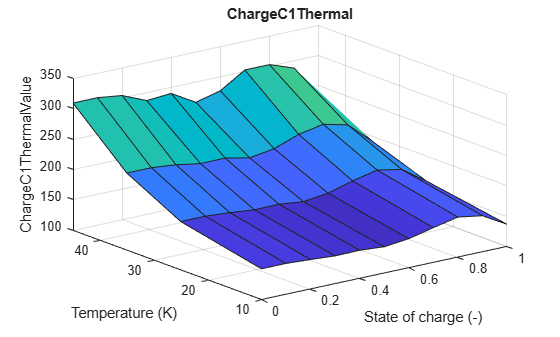The `battModel` workspace variable contains all relevant data for the parameterization and it specifies all the parameters of the Battery (Table-Based) block in the `CellDischargeCC` SLX file. This figure shows the values for each parameter inside the Battery (Table-Based) block.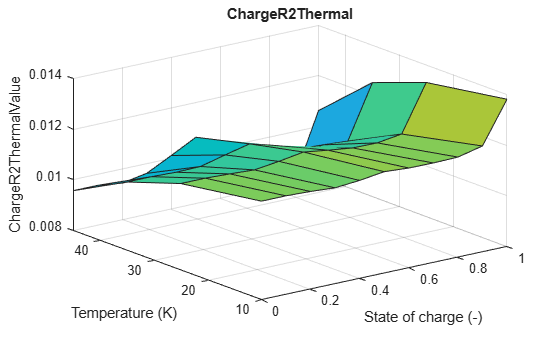Set the minimum and the maximum C-rates for the simulation.

```oneHr = 3600; C_rate_min = 0.25; C_rate_max = 1; C_rate_del = 0.25; numCases = (C_rate_max - C_rate_min)/C_rate_del + 1;```

Set the battery thermal mass, initial temperature, and initial SOC. The temperature of the cell depends on the `CellThermalMass`, which is equal to 200 J/K.

```CellThermalMass = 200; InitialTemperature = 305; InitialSOC = 1;```

Run a constant current discharge at different C rates, for a given `InitialTemperature`.

```legendStr = strings(1,numCases); battSensorData = cell(1,numCases);```

Run all simulations:

```caseNum = 0; for C_rate = C_rate_min:C_rate_del:C_rate_max caseNum = caseNum + 1; legendStr(1,caseNum) = strcat(num2str(C_rate),'C rate discharge case'); BatteryConstantCurrent = C_rate*battModel.CellCapacityAhr; batterySimTime_s = oneHr/C_rate; sim('CellDischargeCC'); ts = simlog_CellDischargeCC.battery.v.series.time; voltage = simlog_CellDischargeCC.battery.v.series.values; tempK = simlog_CellDischargeCC.battery.cell_temperature.series.values; battSensorData{1,caseNum} = [ts voltage tempK]; end```

Plot the battery voltage for different constant current discharge cases:

```titleDisplay = strcat('Cell Voltage, Ambient Temperature =',num2str(InitialTemperature-273),' degC'); figure('Name', titleDisplay); for itr = 1:numCases plot(battSensorData{1,itr}(:,1),battSensorData{1,itr}(:,2)); hold on end hold off legend(legendStr); xlabel('Time'); ylabel('Voltage'); title(titleDisplay);```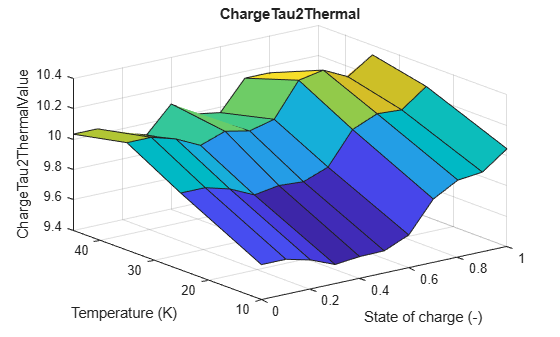Plot the battery temperature rise during constant current discharge cases:

```titleDisplay = strcat('Cell Temperature, Ambient Temperature =',num2str(InitialTemperature-273),' degC'); figure('Name', titleDisplay); for itr = 1:numCases plot(battSensorData{1,itr}(:,1),battSensorData{1,itr}(:,3)); hold on end hold off legend(legendStr); xlabel('Time'); ylabel('Temperature (K)'); title(titleDisplay);```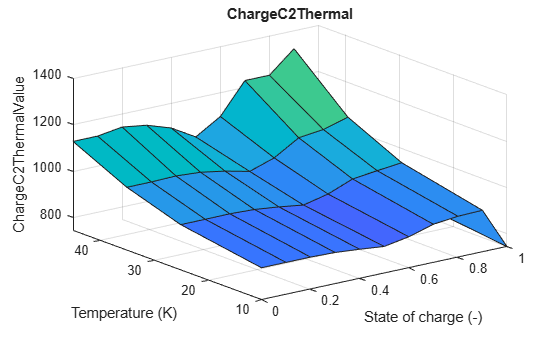### Reference

1. Christophersen, Jon P. Battery Test Manual For Electric Vehicles, Revision 3. United States: N. p., 2015. Web. doi:10.2172/1186745